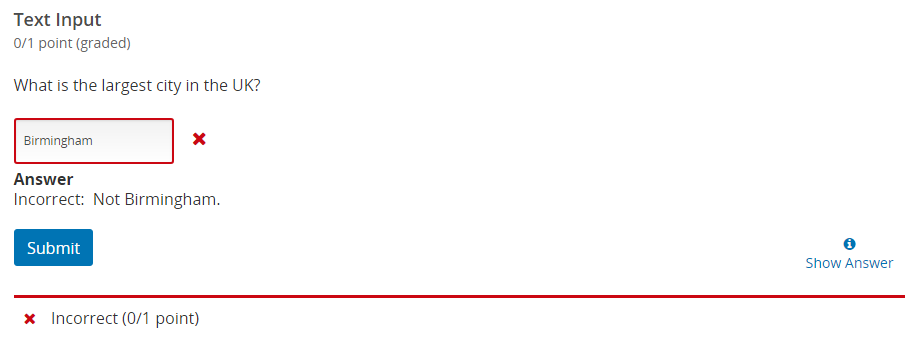Adaptive feedback allows you to present different feedback for different answers to problems in Open edX. For example, you may wish to use this to tell a learner exactly why they may have gotten a particular wrong answer. This type of feedback is available in the following problem types:

• Multiple choice: On all answers
• Checkboxes: On each individual answer, as well as when an option is not selected and when certain combinations of answers are selected
• Numerical input: On the correct answer only (and thus isn't really adaptive, but we'll include it here for completeness)
• Text input: On the correct answers, and on specific incorrect answers.

In this article, we're going to look at how to add adaptive feedback to each one of these input types. The examples given here are used in the simple problem editor. To learn how to insert these problems and use the simple problem editor, try other help articles such as  Adding a problem to your Open edX course.

## Multiple Choice

The syntax for adding adaptive feedback to multiple choice problems is as follows:

```( ) Incorrect answer  {{Label:: Feedback}}
```

The feedback to provide when the learner selects an answer is contained within the curled braces alongside each answer. Note that you don't always need a label on your feedback, you just have to have two colons (:) before your feedback if you do so.

For example:

```>>What is the answer to this question?||Pick the correct answer.<<

( ) an incorrect answer {{Incorrect:: This is not the correct answer, consider getting it right.}}
(x) the correct answer {{Good job! You got it right.}}
```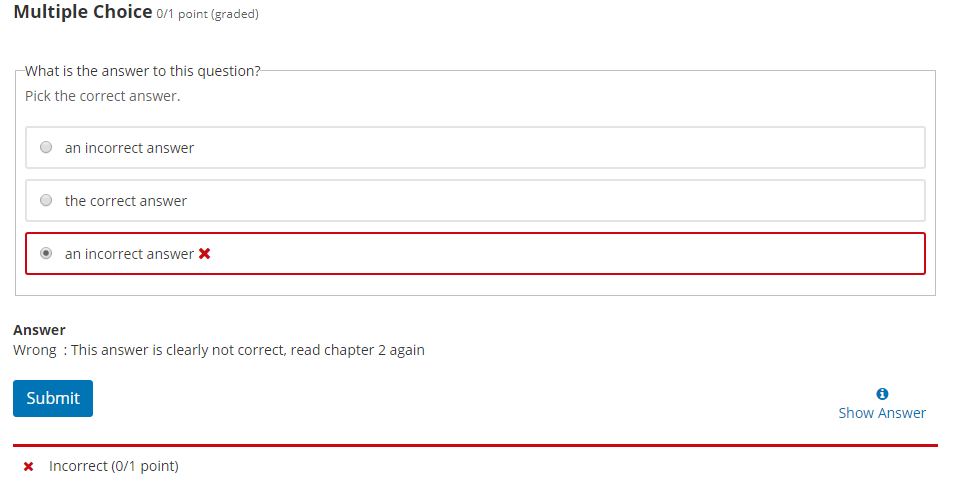## Checkboxes

Checkboxes are very similar to multiple choice, but in this problem type you have to specify whether the feedback appears when the option is selected or when it's unselected, in the following format:

```[ ] Incorrect Answer {{selected: Feedback to display when the answer is selected.},{unselected: Feedback to display when the answer is not selected.}
[x] Correct Answer {{selected: Feedback to display when the answer is selected.}}
[x] Correct Answer {{unselected: Feedback to display when the answer is not selected.}}
```

Note that the placement of the curved braces is important when dealing with multiple sets of feedback for selected/unselected answers. For example:

```>>Which of these answers is correct?||Pick three.<<

[x] This one {{unselected: You missed the first one.}}
[ ] Not this one {{selected: The second one is wrong.}}
[ ] Also not this one {{selected: We explicitly said not the third one.}, {unselected: Good job not picking one of the wrong answers.}}
[x] Definitely this one {{selected: The fourth one is correct.}}
```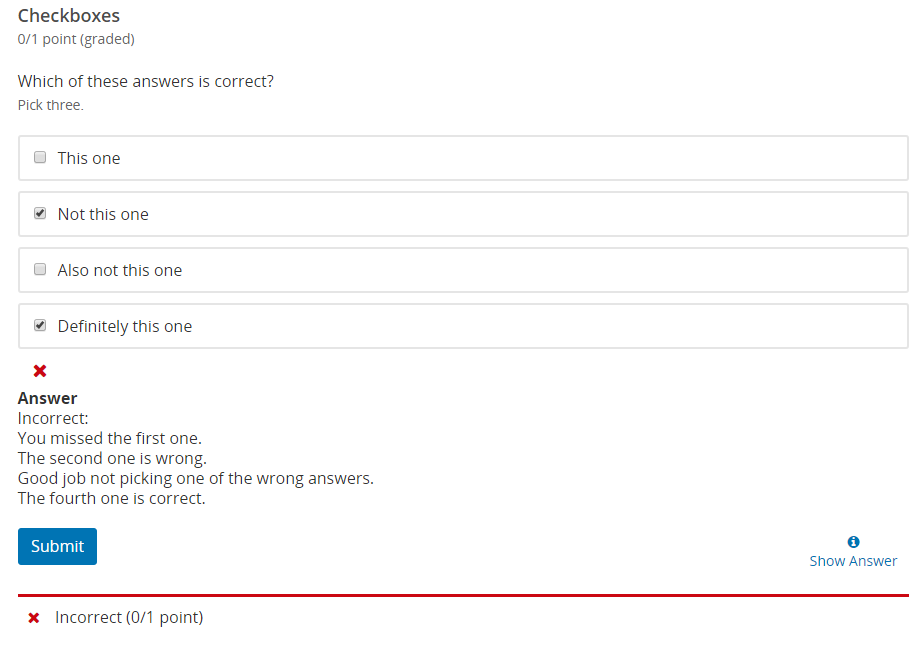The second way to provide feedback on this sort of problem is to use letter references to refer to groups of answers, AKA compound feedback. In the case of the example above, we add in some extra lines at the bottom with the following structure:

```{{((A B C D)) Feedback for selection of this combination of answers.}}
```

Note that compound feedback overrides all other feedback, including feedback for answers that are not selected, and is only shown in the event of their selection exactly matching (for example if the feedback is given for answers A and B, a learner who selects answers A, B and C will not receive the compound feedback). You can also have as many of these as you want, to account for different answer combinations. Applying a single piece of compound feedback to our example, we get the following:

```>>Which of these answers is correct?||Pick three.<<
[x] This one {{unselected: You missed the first one.}}
[ ] Not this one {{selected: The second one is wrong.}}
[ ] Also not this one {{selected: We explicitly said not the third one.}, {unselected: Good job not picking one of the wrong answers.}}
[x] Definitely this one {{selected: The fourth one is correct.}}
{{((B D)) You picked B and D. B is wrong.}}
```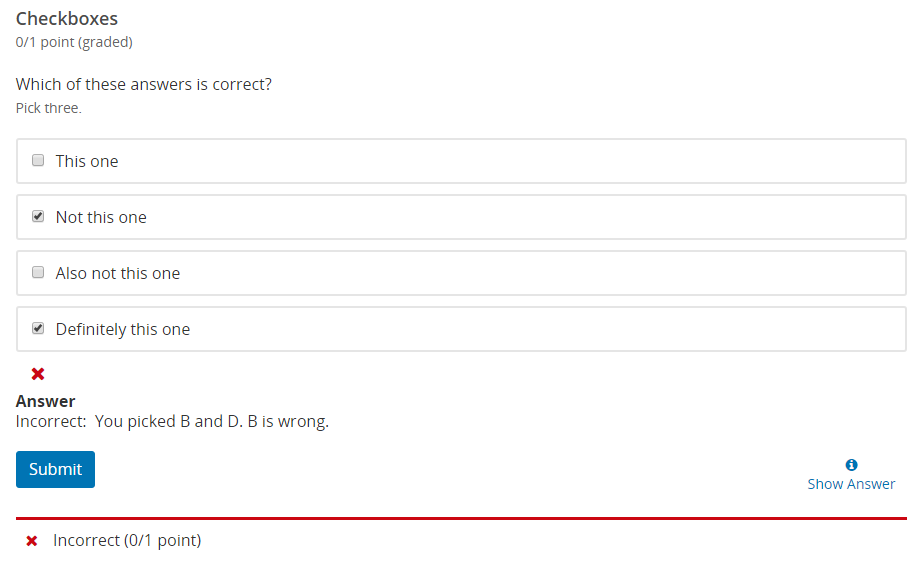## Numerical Input

Numerical input problems only support feedback on the correct answer. As previously mentioned, this means it's not adaptive like the others, but we'll cover it anyway. To add it, you simply put it in curled braces after the answer line, similarly to multiple choice:

```=answer {{Feedback to give when the answer is correct.}}
```

In practice, this looks like this:

```>>What's 10x10?<<
= 100 {{Good job on knowing basic multiplication}}
```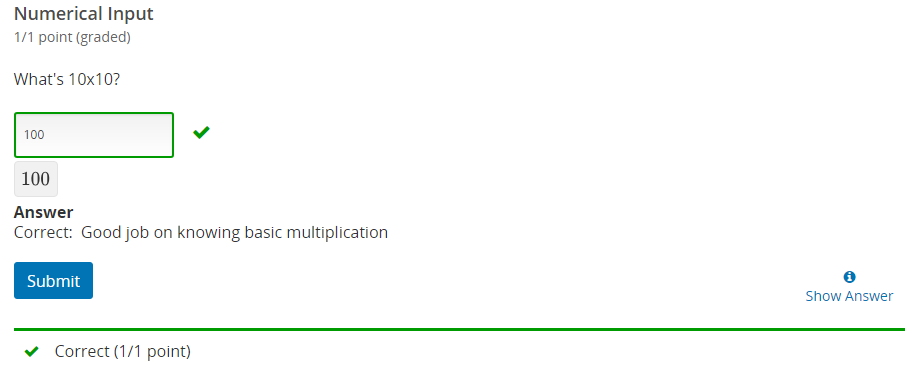## Text input

Single line text input problems support feedback on the correct answer, but also on specific incorrect answers. This is useful for common misconceptions. The basic format for these is as follows:

```>>Question<<
= the correct answer {{Feedback that is shown when this answer is given}}
or= optional acceptable variant of the correct answer {{Feedback that is shown when this answer is given}}
not= optional incorrect answer such as a frequent misconception  {{Feedback that is shown when this answer is given}}
```

You can include as may "or=" or "not=" lines with their own feedback as you wish. In practice, this looks like this:

```>>What is the largest city in the UK?<<
= London {{Correct, London is huge in both population and area.}}
or= Londinium {{While this hasn't been the correct name of London since the Romans left in the first century, in this case I'll allow it.}}
not= Birmingham {{Not Birmingham.}}
not= Milton Keynes {{Milton Keynes isn't even technically a city.}}
```